# RD Sharma Solutions for Class 10 Maths Chapter 3 Pair of Linear Equations In Two Variables Exercise 3.7

Another application of linear equations in two variables is solving problems based on numbers. The RD Sharma Solutions Class 10 created by experts at BYJU’S can serve as an important tool for reference and preparing for exams. Students wishing to get a good grip on this particular application of LPE can utilise the RD Sharma Solutions for Class 10 Maths Chapter 3 Pair Of Linear Equations In Two Variables Exercise 3.7 PDF given below.

## RD Sharma Solutions for Class 10 Maths Chapter 3 Pair of Linear Equations In Two Variables Exercise 3.7 Download PDF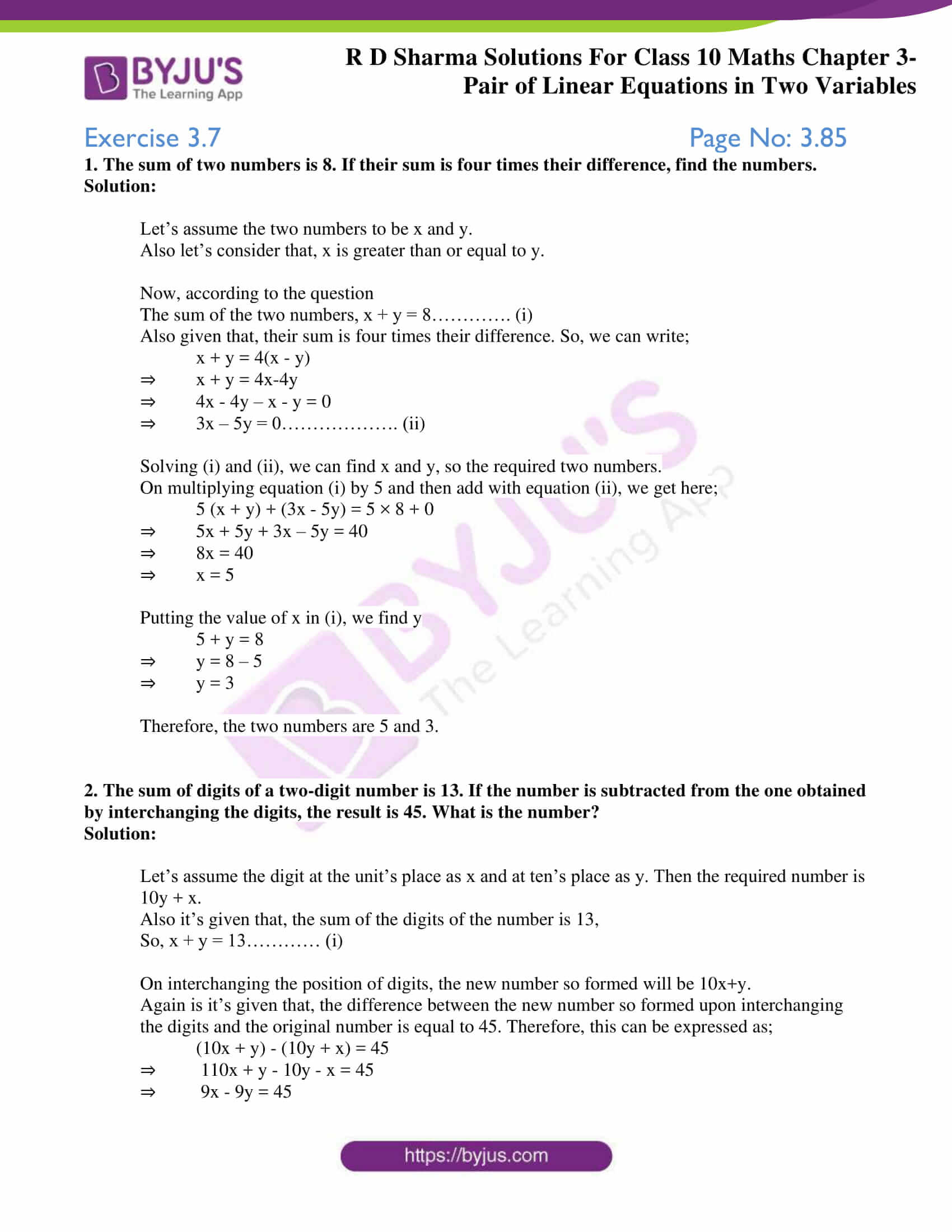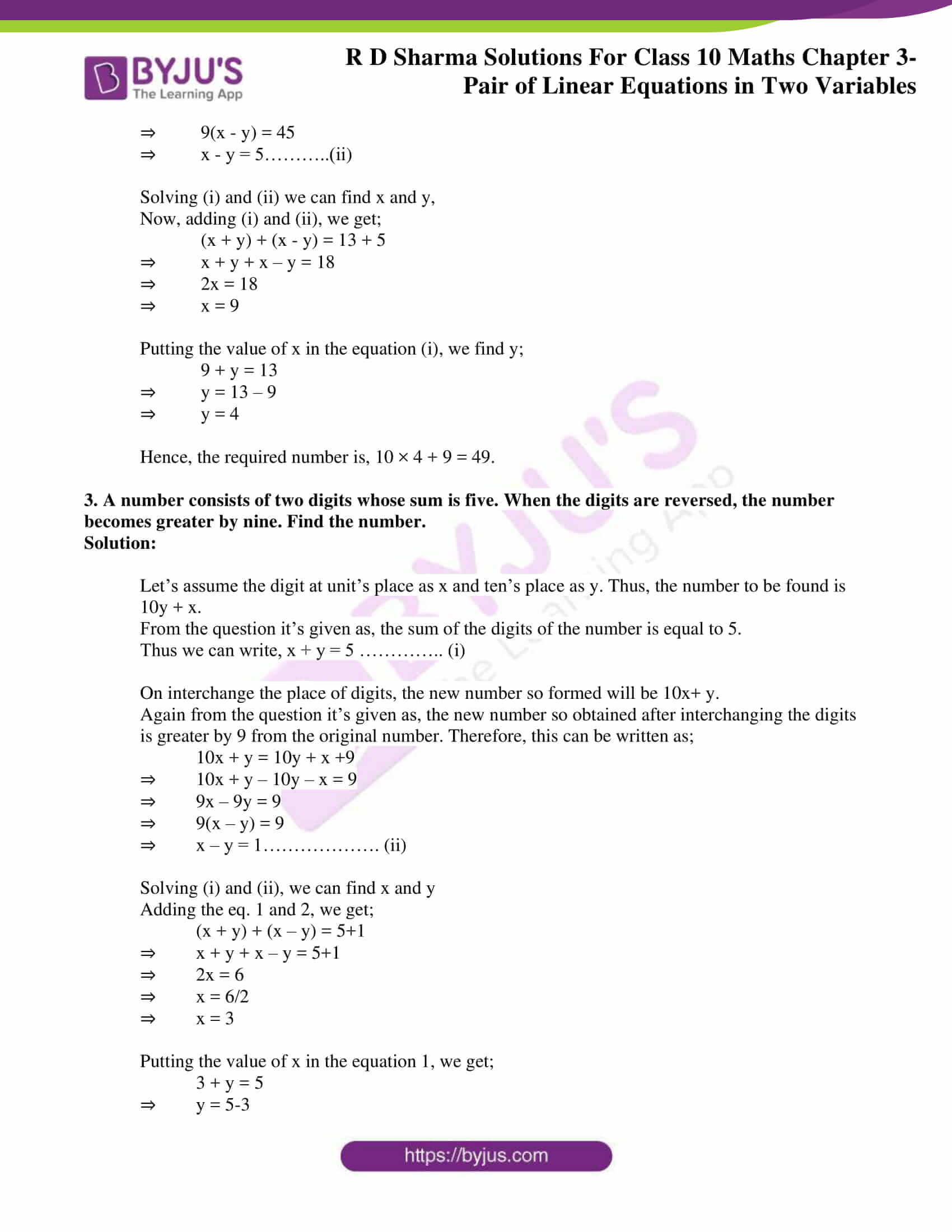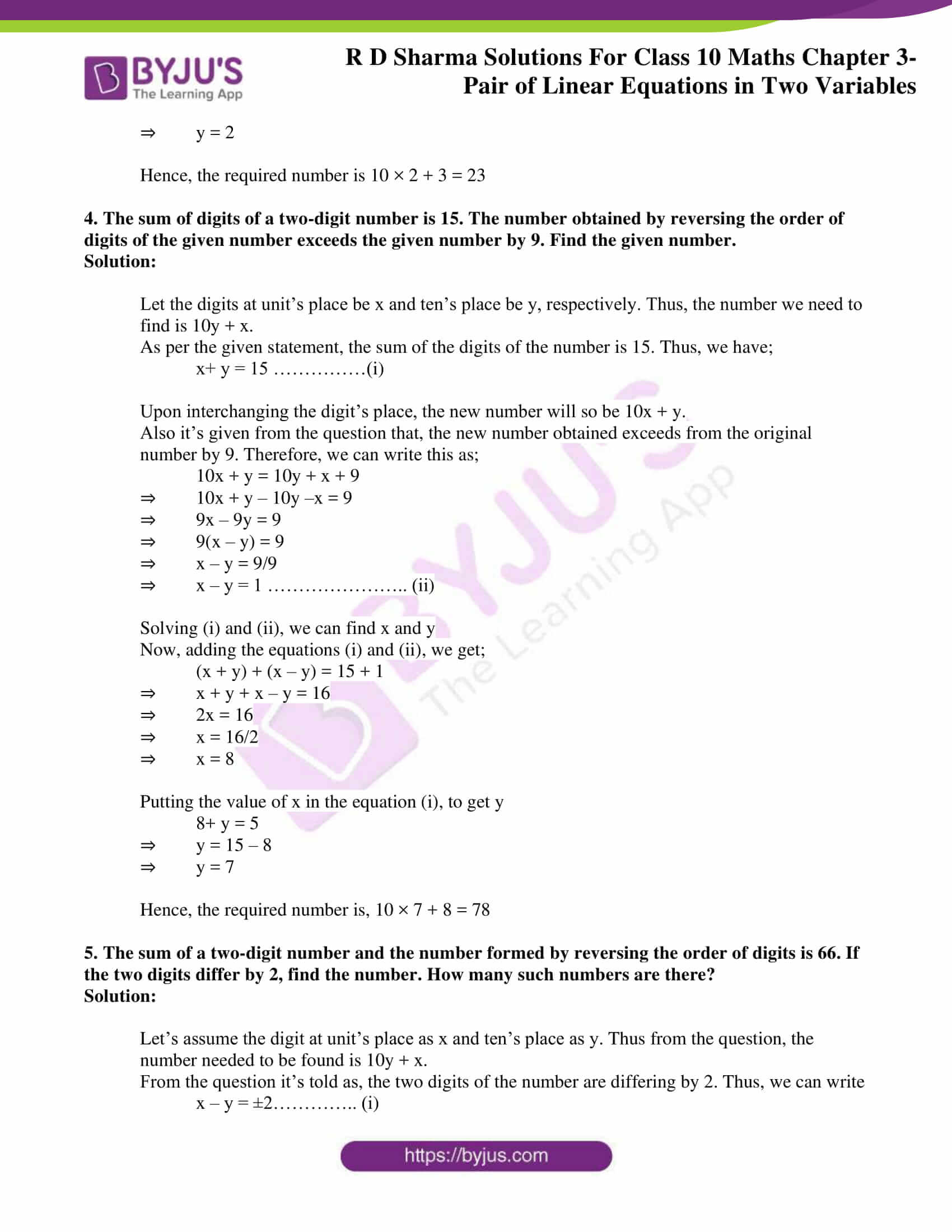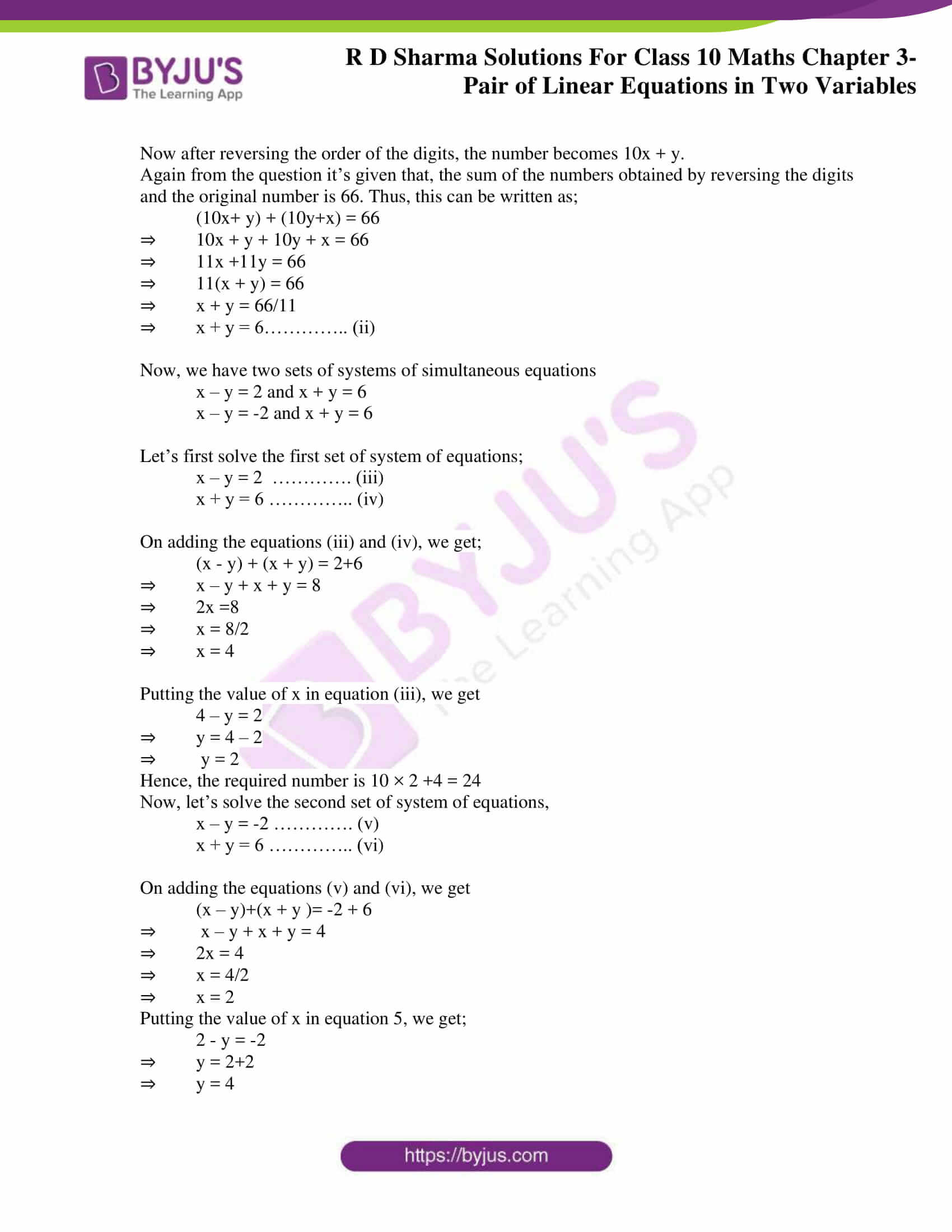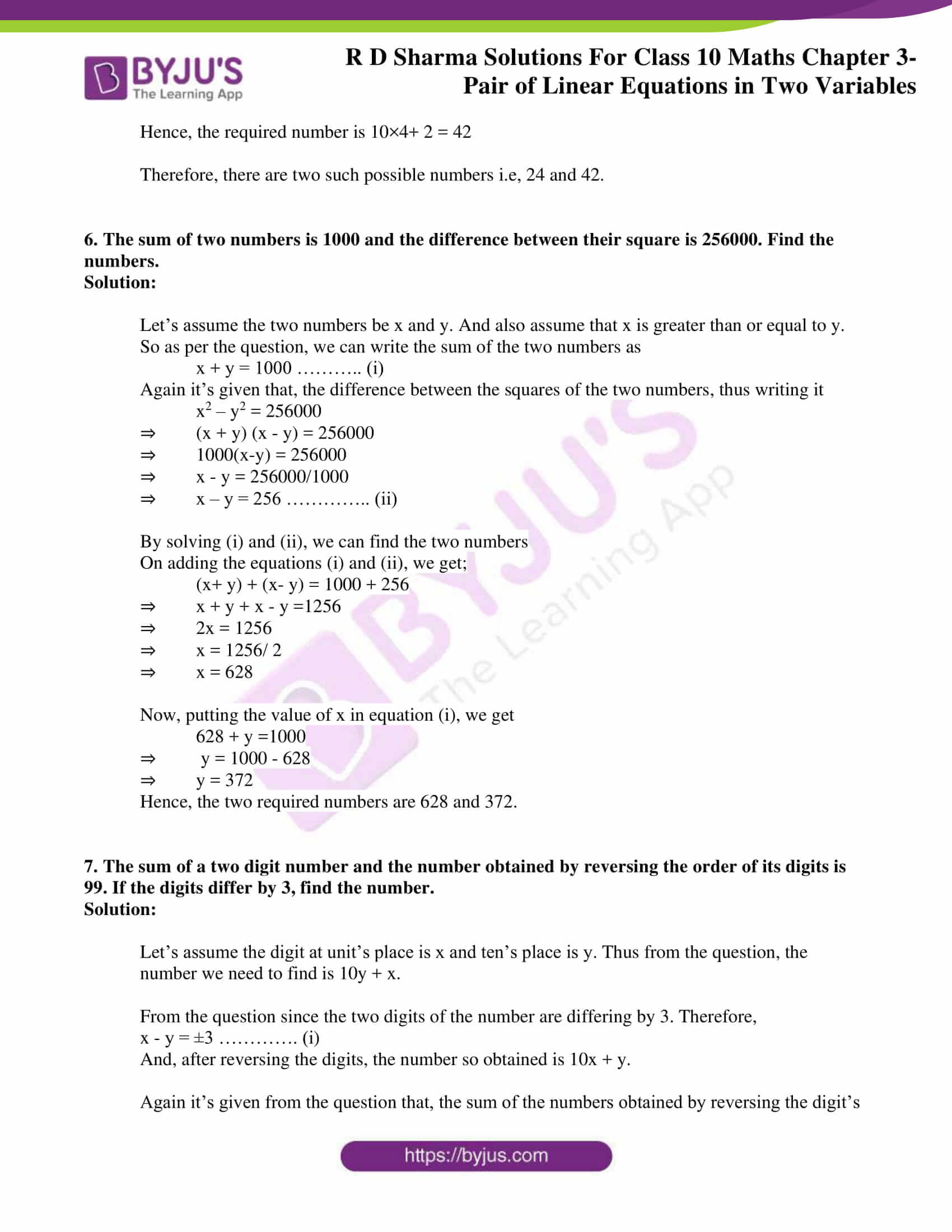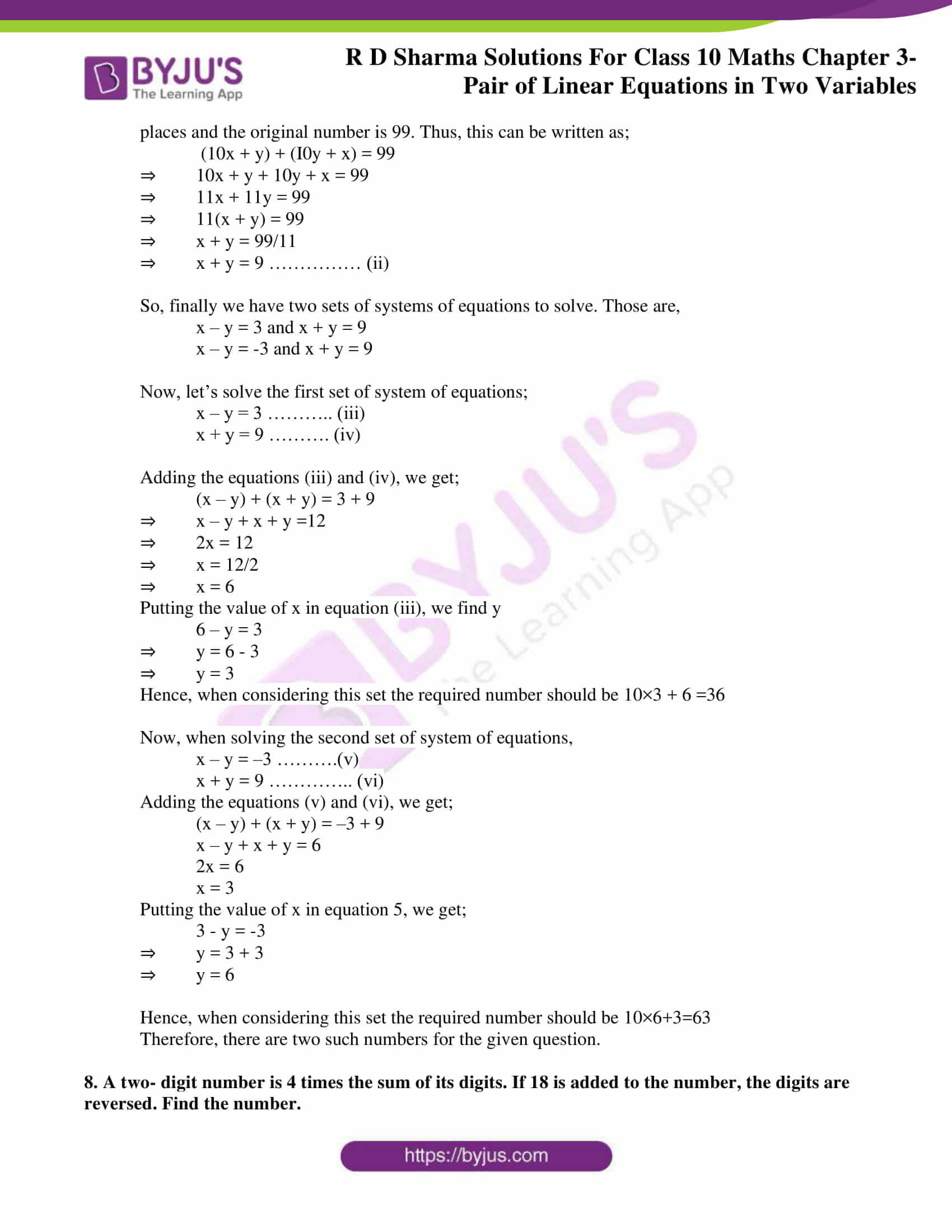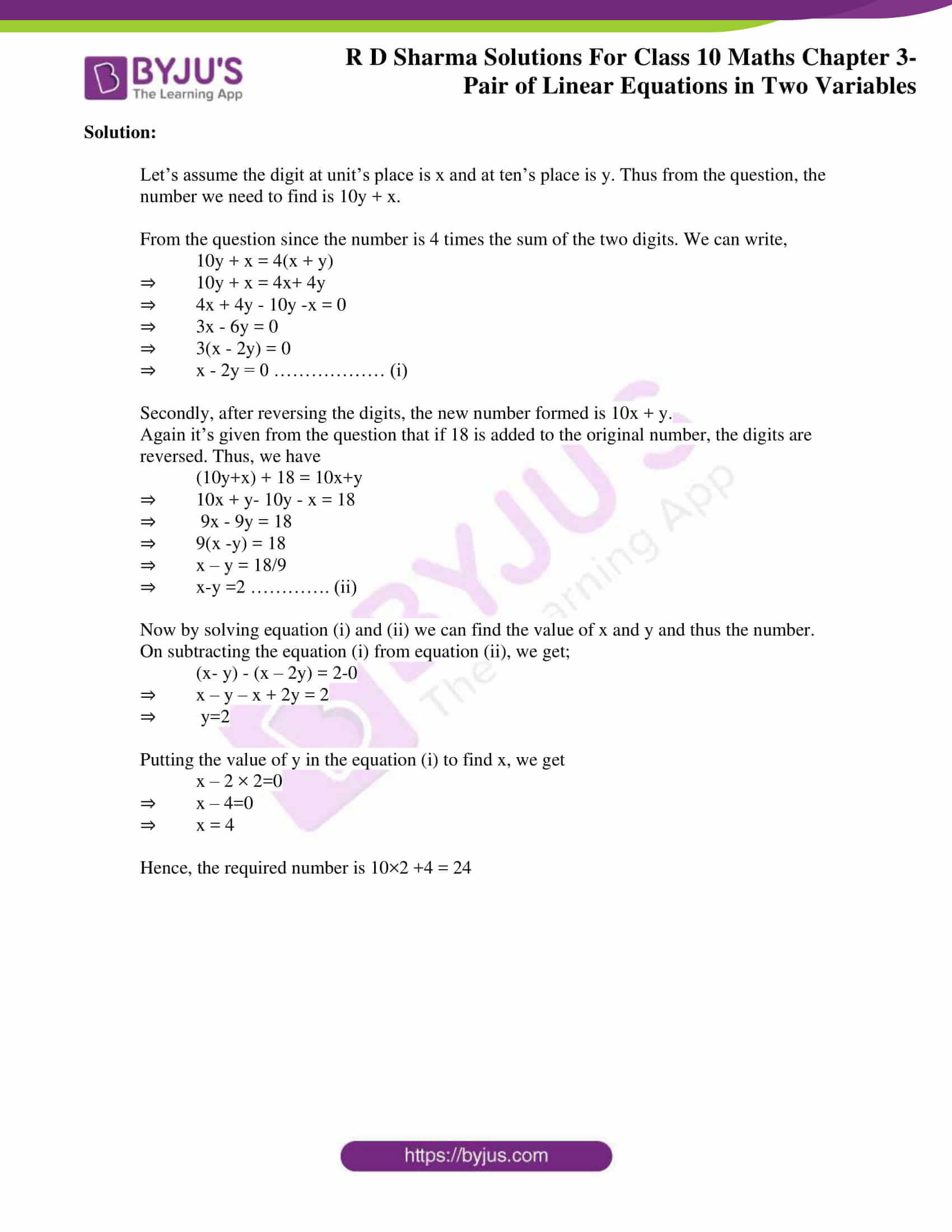### Access RD Sharma Solutions for Class 10 Maths Chapter 3 Pair of Linear Equations In Two Variables Exercise 3.7

1. The sum of two numbers is 8. If their sum is four times their difference, find the numbers.

Solution:

Let’s assume the two numbers to be x and y.

Also let’s consider that, x is greater than or equal to y.

Now, according to the question

The sum of the two numbers, x + y = 8…………. (i)

Also given that, their sum is four times their difference. So, we can write;

x + y = 4(x – y)

⇒ x + y = 4x-4y

⇒ 4x – 4y – x – y = 0

⇒ 3x – 5y = 0………………. (ii)

Solving (i) and (ii), we can find x and y, so the required two numbers.

On multiplying equation (i) by 5 and then add with equation (ii), we get here;

5 (x + y) + (3x – 5y) = 5 × 8 + 0

⇒ 5x + 5y + 3x – 5y = 40

⇒ 8x = 40

⇒ x = 5

Putting the value of x in (i), we find y

5 + y = 8

⇒ y = 8 – 5

⇒ y = 3

Therefore, the two numbers are 5 and 3.

2. The sum of digits of a two-digit number is 13. If the number is subtracted from the one obtained by interchanging the digits, the result is 45. What is the number?

Solution:

Let’s assume the digit at the unit’s place as x and at ten’s place as y. Then the required number is 10y + x.

Also it’s given that, the sum of the digits of the number is 13,

So, x + y = 13………… (i)

On interchanging the position of digits, the new number so formed will be 10x+y.

Again is it’s given that, the difference between the new number so formed upon interchanging the digits and the original number is equal to 45. Therefore, this can be expressed as;

(10x + y) – (10y + x) = 45

⇒ 110x + y – 10y – x = 45

⇒ 9x – 9y = 45

⇒ 9(x – y) = 45

⇒ x – y = 5………..(ii)

Solving (i) and (ii) we can find x and y,

Now, adding (i) and (ii), we get;

(x + y) + (x – y) = 13 + 5

⇒ x + y + x – y = 18

⇒ 2x = 18

⇒ x = 9

Putting the value of x in the equation (i), we find y;

9 + y = 13

⇒ y = 13 – 9

⇒ y = 4

Hence, the required number is, 10 × 4 + 9 = 49.

3. A number consists of two digits whose sum is five. When the digits are reversed, the number becomes greater by nine. Find the number.

Solution:

Let’s assume the digit at unit’s place as x and ten’s place as y. Thus, the number to be found is 10y + x.

From the question it’s given as, the sum of the digits of the number is equal to 5.

Thus we can write, x + y = 5 ………….. (i)

On interchange the place of digits, the new number so formed will be 10x+ y.

Again from the question it’s given as, the new number so obtained after interchanging the digits is greater by 9 from the original number. Therefore, this can be written as;

10x + y = 10y + x +9

⇒ 10x + y – 10y – x = 9

⇒ 9x – 9y = 9

⇒ 9(x – y) = 9

⇒ x – y = 1………………. (ii)

Solving (i) and (ii), we can find x and y

Adding the eq. 1 and 2, we get;

(x + y) + (x – y) = 5+1

⇒ x + y + x – y = 5+1

⇒ 2x = 6

⇒ x = 6/2

⇒ x = 3

Putting the value of x in the equation 1, we get;

3 + y = 5

⇒ y = 5-3

⇒ y = 2

Hence, the required number is 10 × 2 + 3 = 23

4. The sum of digits of a two-digit number is 15. The number obtained by reversing the order of digits of the given number exceeds the given number by 9. Find the given number.

Solution:

Let the digits at unit’s place be x and ten’s place be y, respectively. Thus, the number we need to find is 10y + x.

As per the given statement, the sum of the digits of the number is 15. Thus, we have;

x+ y = 15 ……………(i)

Upon interchanging the digit’s place, the new number will so be 10x + y.

Also it’s given from the question that, the new number obtained exceeds from the original number by 9. Therefore, we can write this as;

10x + y = 10y + x + 9

⇒ 10x + y – 10y –x = 9

⇒ 9x – 9y = 9

⇒ 9(x – y) = 9

⇒ x – y = 9/9

⇒ x – y = 1 ………………….. (ii)

Solving (i) and (ii), we can find x and y

Now, adding the equations (i) and (ii), we get;

(x + y) + (x – y) = 15 + 1

⇒ x + y + x – y = 16

⇒ 2x = 16

⇒ x = 16/2

⇒ x = 8

Putting the value of x in the equation (i), to get y

8+ y = 5

⇒ y = 15 – 8

⇒ y = 7

Hence, the required number is, 10 × 7 + 8 = 78

5. The sum of a two-digit number and the number formed by reversing the order of digits is 66. If the two digits differ by 2, find the number. How many such numbers are there?

Solution:

Let’s assume the digit at unit’s place as x and ten’s place as y. Thus from the question, the number needed to be found is 10y + x.

From the question it’s told as, the two digits of the number are differing by 2. Thus, we can write

x – y = ±2………….. (i)

Now after reversing the order of the digits, the number becomes 10x + y.

Again from the question it’s given that, the sum of the numbers obtained by reversing the digits and the original number is 66. Thus, this can be written as;

(10x+ y) + (10y+x) = 66

⇒ 10x + y + 10y + x = 66

⇒ 11x +11y = 66

⇒ 11(x + y) = 66

⇒ x + y = 66/11

⇒ x + y = 6………….. (ii)

Now, we have two sets of systems of simultaneous equations

x – y = 2 and x + y = 6

x – y = -2 and x + y = 6

Let’s first solve the first set of system of equations;

x – y = 2  …………. (iii)

x + y = 6 ………….. (iv)

On adding the equations (iii) and (iv), we get;

(x – y) + (x + y) = 2+6

⇒ x – y + x + y = 8

⇒ 2x =8

⇒ x = 8/2

⇒ x = 4

Putting the value of x in equation (iii), we get

4 – y = 2

⇒ y = 4 – 2

⇒ y = 2

Hence, the required number is 10 × 2 +4 = 24

Now, let’s solve the second set of system of equations,

x – y = -2 …………. (v)

x + y = 6 ………….. (vi)

On adding the equations (v) and (vi), we get

(x – y)+(x + y )= -2 + 6

⇒ x – y + x + y = 4

⇒ 2x = 4

⇒ x = 4/2

⇒ x = 2

Putting the value of x in equation 5, we get;

2 – y = -2

⇒ y = 2+2

⇒ y = 4

Hence, the required number is 10×4+ 2 = 42

Therefore, there are two such possible numbers i.e, 24 and 42.

6. The sum of two numbers is 1000 and the difference between their square is 256000. Find the numbers.

Solution:

Let’s assume the two numbers be x and y. And also assume that x is greater than or equal to y.

So as per the question, we can write the sum of the two numbers as

x + y = 1000 ……….. (i)

Again it’s given that, the difference between the squares of the two numbers, thus writing it

x2 – y2 = 256000

⇒ (x + y) (x – y) = 256000

⇒ 1000(x-y) = 256000

⇒ x – y = 256000/1000

⇒ x – y = 256 ………….. (ii)

By solving (i) and (ii), we can find the two numbers

On adding the equations (i) and (ii), we get;

(x+ y) + (x- y) = 1000 + 256

⇒ x + y + x – y =1256

⇒ 2x = 1256

⇒ x = 1256/ 2

⇒ x = 628

Now, putting the value of x in equation (i), we get

628 + y =1000

⇒ y = 1000 – 628

⇒ y = 372

Hence, the two required numbers are 628 and 372.

7. The sum of a two digit number and the number obtained by reversing the order of its digits is 99. If the digits differ by 3, find the number.

Solution:

Let’s assume the digit at unit’s place is x and ten’s place is y. Thus from the question, the number we need to find is 10y + x.

From the question since the two digits of the number are differing by 3. Therefore,

x – y = ±3 …………. (i)

And, after reversing the digits, the number so obtained is 10x + y.

Again it’s given from the question that, the sum of the numbers obtained by reversing the digit’s places and the original number is 99. Thus, this can be written as;

(10x + y) + (I0y + x) = 99

⇒ 10x + y + 10y + x = 99

⇒ 11x + 11y = 99

⇒ 11(x + y) = 99

⇒ x + y = 99/11

⇒ x + y = 9 …………… (ii)

So, finally we have two sets of systems of equations to solve. Those are,

x – y = 3 and x + y = 9

x – y = -3 and x + y = 9

Now, let’s solve the first set of system of equations;

x – y = 3 ……….. (iii)

x + y = 9 ………. (iv)

Adding the equations (iii) and (iv), we get;

(x – y) + (x + y) = 3 + 9

⇒ x – y + x + y =12

⇒ 2x = 12

⇒ x = 12/2

⇒ x = 6

Putting the value of x in equation (iii), we find y

6 – y = 3

⇒ y = 6 – 3

⇒ y = 3

Hence, when considering this set the required number should be 10×3 + 6 =36

Now, when solving the second set of system of equations,

x – y = –3 ……….(v)

x + y = 9 ………….. (vi)

Adding the equations (v) and (vi), we get;

(x – y) + (x + y) = –3 + 9

x – y + x + y = 6

2x = 6

x = 3

Putting the value of x in equation 5, we get;

3 – y = -3

⇒ y = 3 + 3

⇒ y = 6

Hence, when considering this set the required number should be 10×6+3=63

Therefore, there are two such numbers for the given question.

8. A two- digit number is 4 times the sum of its digits. If 18 is added to the number, the digits are reversed. Find the number.

Solution:

Let’s assume the digit at unit’s place is x and at ten’s place is y. Thus from the question, the number we need to find is 10y + x.

From the question since the number is 4 times the sum of the two digits. We can write,

10y + x = 4(x + y)

⇒ 10y + x = 4x+ 4y

⇒ 4x + 4y – 10y -x = 0

⇒ 3x – 6y = 0

⇒ 3(x – 2y) = 0

⇒ x – 2y = 0 ……………… (i)

Secondly, after reversing the digits, the new number formed is 10x + y.

Again it’s given from the question that if 18 is added to the original number, the digits are reversed. Thus, we have

(10y+x) + 18 = 10x+y

⇒ 10x + y- 10y – x = 18

⇒ 9x – 9y = 18

⇒ 9(x -y) = 18

⇒ x – y = 18/9

⇒ x-y =2 …………. (ii)

Now by solving equation (i) and (ii) we can find the value of x and y and thus the number.

On subtracting the equation (i) from equation (ii), we get;

(x- y) – (x – 2y) = 2-0

⇒ x – y – x + 2y = 2

⇒ y=2

Putting the value of y in the equation (i) to find x, we get

x – 2 × 2=0

⇒ x – 4=0

⇒ x = 4

Hence, the required number is 10×2 +4 = 24